by kirupa   |   filed under The Web Corner

Have you ever seen a number that looks like 0x7E0?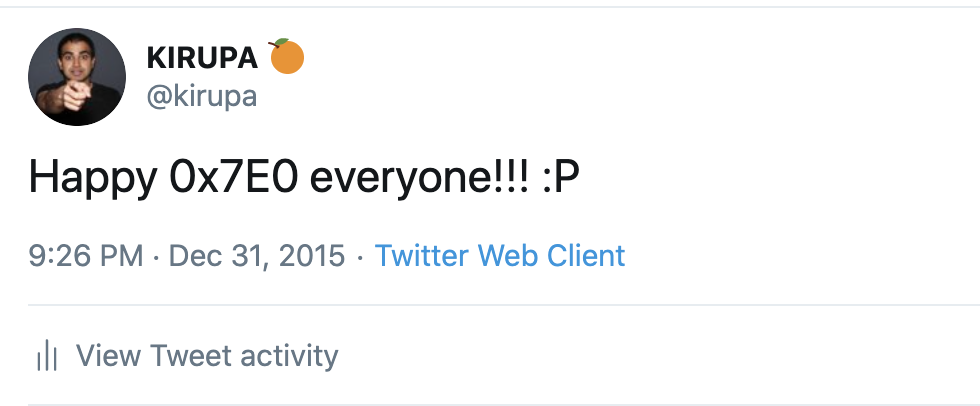How about a color value like #0798FF?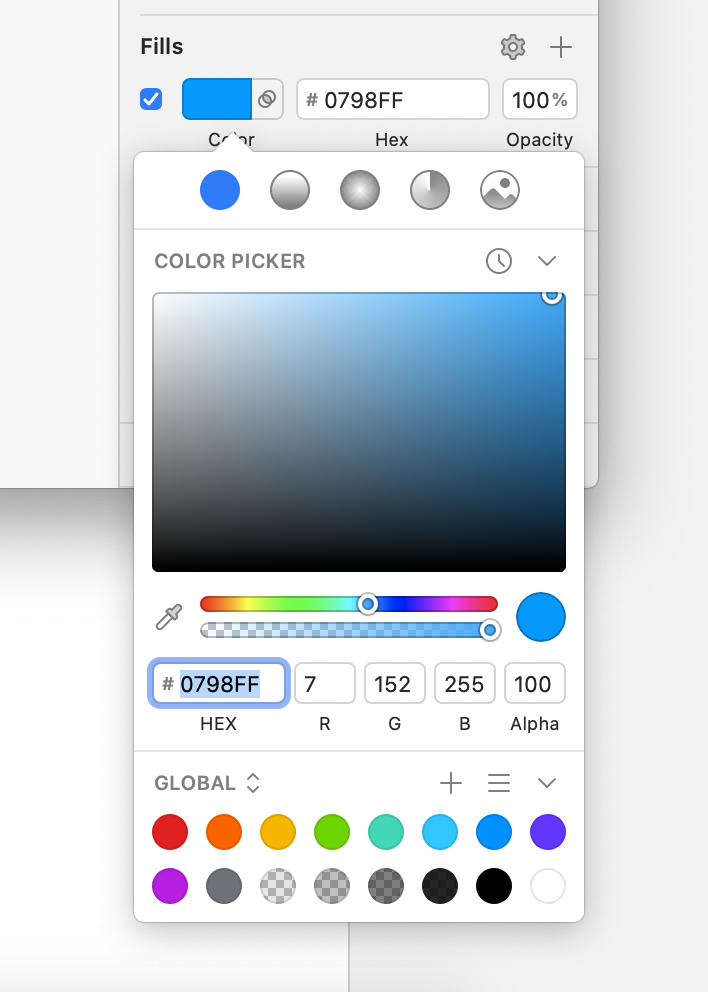If you have (or haven’t - it's all good 😎), what you saw is something known as a hexadecimal number. It’s one of the several popular ways we can represent numbers in a way that our computers can understand. Knowing how to work with these hexadecimal (or just hex or base-16 as the cool kids like to say) numbers is less important today than it used to be. Despite that, it is a useful skill similar to knowing how to start a fire (🔥) using primitive tools and materials (⚒️). You probably won’t use this skill every day or year, but when you do need to use it, you’ll be grateful that you know how. Let’s learn more about hex values in the following sections.

Onwards!

## Let's Look at Normal Base-10/Decimal Numbers

One of the best ways to learn how to count in hex is by looking at how we count normal numbers like the ones we see all the time....normal numbers like 42, 2001, 80, 55, 21, and infinitely more. These normal numbers are more formally known as decimal or base-10 numbers. In a base-10 number scheme, all of our numbers are made up of a combination of 0, 1, 2, 3, 4, 5, 6, 7, 8, 9. Here is where things get a bit bizarre, so bear with me for a moment. In a base-10 world, we can model every number as falling into a region that is divided by powers of 10: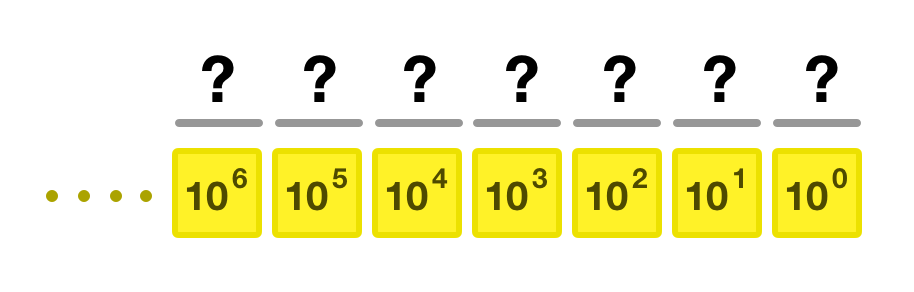That sound cryptic and complicated, but it just means that each part of a base-10 number falls into the 1’s, 10’s, 100’s, 1000’s, or any 10N bucket. Every conceivable base-10 number we want to represent will find itself within a range specified by this model. If we had to represent the number 42, it would look as follows: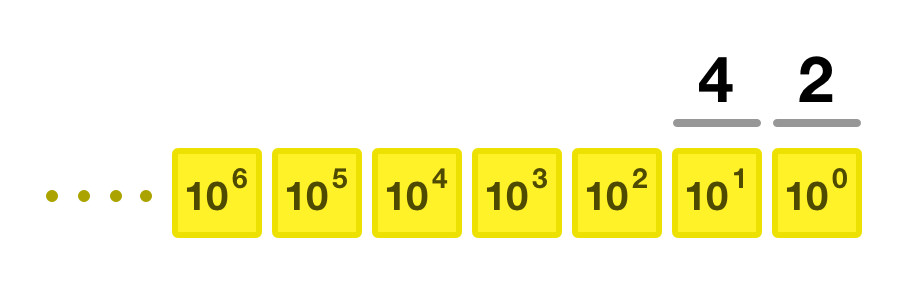That is because 42 is 4 x 101 + 2 x 100. For something larger like 1492, it would be as follows: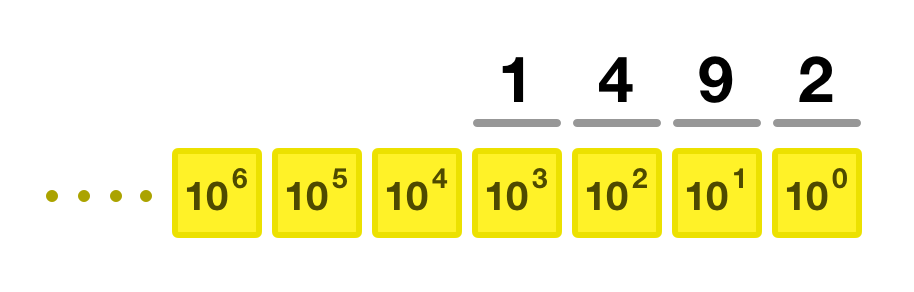1492 is 1 x 103 + 4 x 102 + 9 x 101 + 2 x 100. If we had to go even larger and repeat this for 65536, here is what we would see: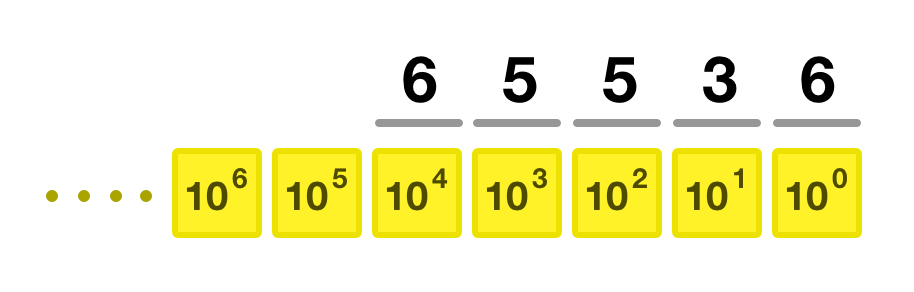The awkward part of all this is that base-10 numbers are easy to represent and make sense of. We don’t need to have these extra layers of visualizations or an expression mapping each number to the appropriate power of 10. We can just plop them down, ensure the smallest digit lines up with 100 and move left until we have no more numbers left. Also, we are going from one base-10 number to the same base-10 number. That greatly simplifies things, to put it lightly 🐤.

When we leave the boring familiarity and easy-ness of base-10 behind and go into more exotic number schemes, that’s where we’ll need to come up with a slightly more general model to go from a decimal based number to one in another number scheme...like base-16!

## A General Model

This general model involves some division and working with a remainder resulting from the division. Let’s look at this model by revisiting our base-10 examples starting with 42. The first thing we do is divide 42 by the base-number, which is 10. The result of this is going to be:

``42 / 10 = 4 remainder 2``

The next step is to divide the 4 (aka the quotient) by 10:

``4 / 10 = 0 remainder 4``

This results in our quotient being 0 and the remainder being 4. At this point, we can’t divide our number any further. The way we can now generate the result is being going backwards and grouping up the remainders. In our case that will be 4 and then 2.

Speeding this up a bit, let’s look at how to calculate the base-10 representation for 1492:

``````1492 / 10 = 149 remainder 2
149 / 10 = 14 remainder 9
14 / 10 = 1 remainder 4
1 / 10 = 0 remainder 1``````

Going backwards and grouping the remainders, we get 1492. For 65536, the calculation looks as follows:

``````65536 / 10 = 6553 remainder 6
6553 / 10 = 655 remainder 3
655 / 10 = 65 remainder 5
65 / 10 = 6 remainder 5
6 / 10 = 0 remainder 6``````

Repeating the same grouping of remainders, the final answer is unsurprisingly 65536. Just like earlier, for base-10 numbers, this general approach isn’t needed as well. We are still taking a base-10 number, doing some calculations, and getting back a base-10 number. In the next section when we dive into hexadecimal numbers, this general model and the previous model we saw will really start to shine.

Now that we’ve looked at the many ways for making sense of base-10 numbers, we are going to shift our focus to the real stars of this show, the hexadecimal / base-16 / hex numbers. In the hex world, the numbers we represent go from 0 to 15. The thing to note is that not all of those numbers are going to be decimals. Numbers 0 through 9 are just base-10 numbers. Once we get to 10, we switch to using letters. Yes, that is right. 10 would be A, 11 would be B, 12 is C, 13 is D, 14 is E, and 15 is F. The mapping between the decimal world and the hexadecimal world for 0 to 15 looks as follows: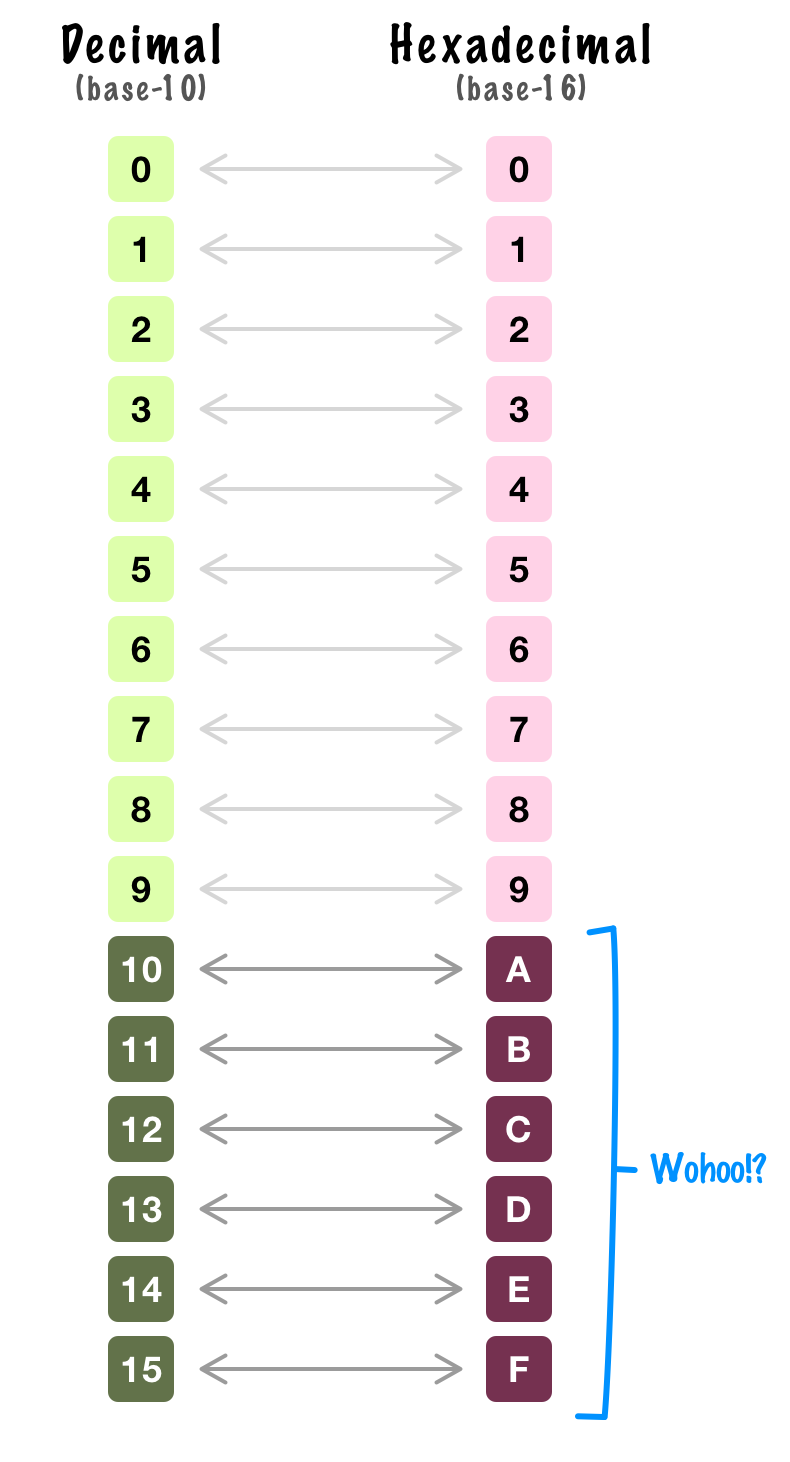Once we move past this difference, the really important task of turning decimal numbers into hex values is just a matter of using the general model we saw earlier.

### Going from Decimal to Hexadecimal

Let’s start by using our general model to go from a decimal value to a hex one. To be consistent, we will kick this party off with 42. Using our general model, the first step will be:

``42 / 16 = 2 remainder 10``

Because we are dealing with a base-16 scheme, notice that we are dividing 42 by 16. The result is a quotient of 2 with a remainder of 10. The next step will be:

``2 / 16 = 0 remainder 2``

All that is left is to combine the remainders, and when we do that, we get 2 and 10. In the hex world, because 10 is represented by an A, the final hex representation for 42 is going to be 2A. In some contexts, a 0x is added in front of the hex value, so 0x2A would be another valid answer.

#### Where does 0x come from?

The 0x is just a constant that is stuck on at the beginning of a hex value. It comes from languages like C where the way you designate a hex number is by adding the 0x in front of the value. Not a very exciting story is it?

Let’s do 1492 next:

``````1492 / 16 = 93 remainder 4
93 / 16 = 5 remainder 13
5 / 16 = 0 remainder 5``````

The hex value would be the combination of 5, 13, and 4. That results in 5D4 (or 0x5D4). The last number we will look at is 65536. That will look like:

``````65536 / 16 = 4096 remainder 0
4096 / 16 = 256 remainder 0
256 / 16 = 16 remainder 0
16 / 16 = 1 remainder 0
1 / 16 = 0 remainder 1``````

The final hex value is then 10000. I know this looks a bit strange, but the math checks out. This might be one of those cases where adding the 0x and having 0x10000 looks a bit more official 🤪

### Going from Hexadecimal to Decimal

We looked at how to go from a decimal number to its equivalent in the hexadecimal world. Now, it’s time for us to go the other way around. We are going to look at how to go from a hex value to a decimal value. Going this direction only involves using some multiplication and addition. Nothing too serious. The best way to see this work is by just diving head first with an example.

We will start with something familiar, 2A. Now, do you remember this arrangement we looked at for decimal numbers?We are going to tweak it a bit and change this to its hexacular base-16 equivalent: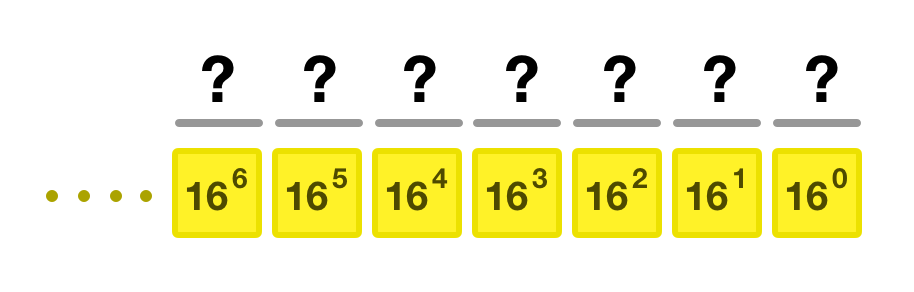Notice that all the powers of 10 are now powers of 16. The first thing we do is slot each digit of our hex value into this arrangement. For 2A, it would be: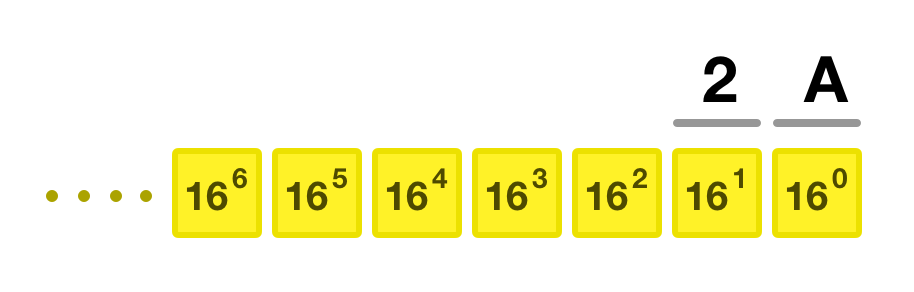Next, we just multiply each value by its position and add it all up:

2 x 161 + 10 x 160 = 42

You don’t need this visualization to calculate the other values. You just need to remember what power each digit of our hex value will map to. For 5D4, the calculation is:

5 x 162 + 13 x 161 + 4 x 160 = 1280 + 208 + 4 = 1492

Similarly, getting the decimal equivalent of 10000 in hex is just:

1 x 164 + 0 x 163 + 0 x 162 + 0 x 161 + 0 x 160 = ⏎
65536 + 0 + 0 + 0 + 0 = 65536

And with this, you are set! You now have all thet tools and tricks necessary to go between decimal and hexadecimal like a pro.

## Conclusion

Well, there you have it. If you ever run into a hexadecimal in the wild, you know exactly how to react:

Now, I don't know about you, but I'm always on the lookout for some number scheme-related trivia. Word on the street is that the famous programmer Charles Simonyi prefers base -2 when interviewing candidates. I don’t even know where to begin with that one, but our generalized model will help you out even in this situation.

Got a question or just want to chat? Comment below or drop by our forums (they are actually the same thing!) where a bunch of the friendliest people you'll ever run into will be happy to help you out!